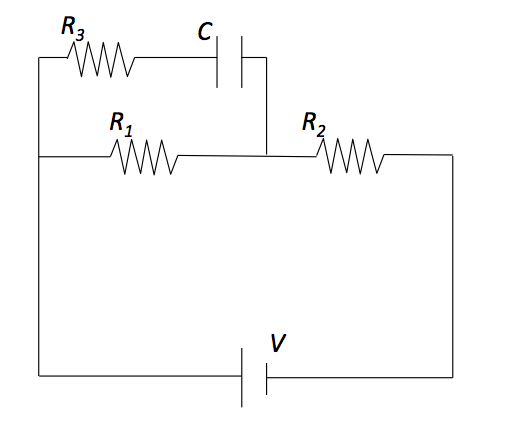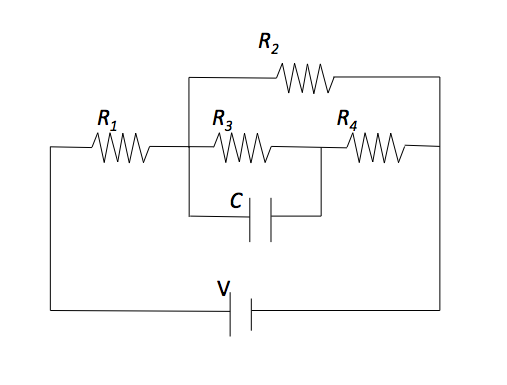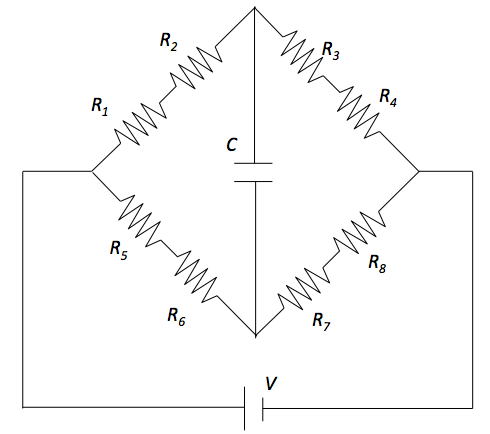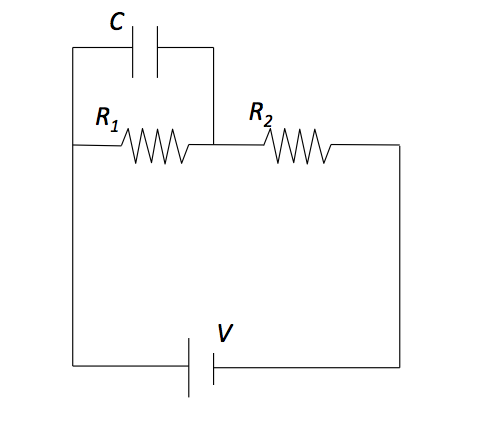Electricity and Magnetism

# RC circuits (direct current)Find the charge on the capacitor in the circuit shown in the figure, at time $t=\infty$. The capacitance of the capacitor is $C= 2 \text{ F},$ resistances of resistors are given by $R_1 = 2 \ \Omega,$ $R_2 = 2 \ \Omega,$ and $R_3 = 5 \ \Omega$ and the voltage of the ideal battery is given by $V= 35 \text{ V}.$Given $R_1 = 3.0\ \Omega,$ $R_2 = 6.0\ \Omega,$ $R_3 = 4.0\ \Omega,$ $R_4 = 8.0\ \Omega.$ Find the charge of the capacitor in the circuit shown in the above figure. The capacitance of the capacitor is $C= 1 \text{ F},$ and the voltage of the ideal battery is given by $V= 21 \text{ V}.$Given $R_1 = 3.0\ \Omega,$ $R_2 = 6.0\ \Omega,$ $R_3 = 6.0\ \Omega,$ $R_4 = 12.0\ \Omega,$ $R_5 = 8.0\ \Omega,$ $R_6 = 4.0\ \Omega,$ $R_7 = 2.0\ \Omega$ and $R_8 = 4.0\ \Omega.$ Find the charge of the capacitor in the circuit shown in the figure. The capacitance of the capacitor is $C= 2 \text{ F},$ and the voltage of the ideal battery is given by $V= 54 \text{ V}.$Find the charge of the capacitor in the circuit shown in the figure above. The capacitance of the capacitor is $C= 4 \text{ F},$ the resistances of the resistors are $R_1 = 2 \ \Omega$ and $R_2 = 5 ,\ \Omega$ and the voltage of the ideal battery is given by $V= 35 \text{ V}.$Given $R_1 = 2.0\ \Omega,$ $R_2 = 4.0\ \Omega,$ $R_3 = 7.0\ \Omega,$ $R_4 = 14.0\ \Omega,$ $R_5 = 6.0\ \Omega,$ $R_6 = 3.0\ \Omega,$ $R_7 = 1.0\ \Omega$ and $R_8 = 2.0\ \Omega.$ Find the charge of the capacitor in the circuit shown in the figure. The capacitance of the capacitor is $C= 1 \text{ F},$ and the voltage of the ideal battery is given by $V= 36 \text{ V}.$

×